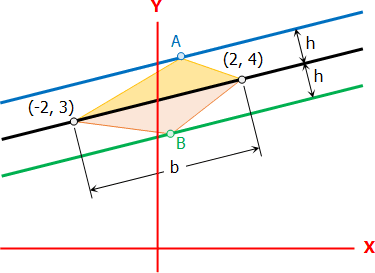# Analytic Geometry Problem Set [Locked: Multiple Questions]

5 posts / 0 new
TungstenAnalytic Geometry Problem Set [Locked: Multiple Questions]

Analytic Geometry Problem Set

I really need help in solving this problem I don't how to do some of it. Please.

Problem 1:
A line passes through point (2,2). Find the equation of the line if the length of the line segment intercepted by the coordinate axes is the square root of 5.

Problem 2:
What is the equation of the line bisecting the bigger angle formed by the intersection of the lines 2x-y+2=0 and 2x+y-4=0?

Problem 3:
The points P(-3, 6), Q(1,-5), R(7, 2) are vertices of a triangle. Find the interior angles.

Problem 4:
The two points on the lines 2x + 3y + 4 = 0 which are at distance 4 from the line 3x + 4y – 6 = 0 are?

Problem 5:
A circle is circumscribing a triangle formed by the lines y = 0, y = x, and 2x + 3y = 10. Find the area of the circle in square units.

Problem 6:
A chord of the circle x2+y2+4x+6y-32=0 is 12 units long. How far is it from the center of the circle?

Problem 7:
Two vertices of a triangle are (2,4) and (-2,3) and the area is 2 sq. units, the equation of the line in which the third vertex belongs is?

Problem 8:
Determine the locus of a point that moves so that the sum of the squares of its distances from the lines 5x + 12y – 4 = 0 and 12x – 5y + 10 = 0 is 5.

Problem 9:
A circle has an equation of x^2 + y^2 + 6x + 6y + 8 = 0 and a line x – 2y + 2 = 0 divides the circle into two parts. Find the equations of the tangent lines at points of intersection (P1 & P2). What is the area the smallest part or division.

Problem 10:
A point moves such that the sum of any point of the curve from two points whose coordinates are (-10,-2) and (6,-2) is always equal to 20 units. Determine what type of conic section formed. Locate the center, the foci, the axes, and the endpoints of the latera recta (if any). Compute its area and perimeter

Abigail Dela CruzHow to solve problem #7?

Jhun VertTwo vertices of a triangle are (2,4) and (-2,3) and the area is 2 sq. units, the equation of the line in which the third vertex belongs is?

Distance between (2, 4) and (-2, 3)
$b = \sqrt{(-2 - 2)^2 + (3 - 4)^2} = \sqrt{17}$

Altitude of the triangle:
$A = \frac{1}{2}bh$

$2 = \frac{1}{2}\sqrt{17} h$

$h = \dfrac{4}{\sqrt{17}}$

The required line is parallel to the line that passes through (2,4) and (-2,3) and at a distance h from it. There are two such lines, one shown in blue color and the other in green. From the figure, point A can be anywhere on the blue line and B and can be any point on the green line.Equation of the line through (2,4) and (-2,3) - black line
$\dfrac{y - 4}{x - 2} = \dfrac{3 - 4}{-2 - 2}$

$y - 4 = \frac{1}{4}(x - 2)$

$4y - 16 = x - 2$

$x - 4y + 14 = 0$

From the formula for distance between parallel lines:
$d = \dfrac{C_2 - C_1}{\pm \sqrt{A^2 + B^2}} = h$

$\dfrac{14 - C_1}{\pm \sqrt{1^2 + 4^2}} = \dfrac{4}{\sqrt{17}}$

$14 - C_1 = \pm 4$

$C_1 = 14 \mp 4$

$C_1 = 10 ~ \text{and} ~ 18$

Answer: x - 4y + 10 = 0 and x - 4y + 18 = 0
Question: From the Answer, which one is the blue line and which one is the green? Comment your answer if you find it out.

Allyssa May CruzHow to solve number 10 ?

Jong Francohow to solve Problem 9

• Mathematics inside the configured delimiters is rendered by MathJax. The default math delimiters are $$...$$ and $...$ for displayed mathematics, and $...$ and $...$ for in-line mathematics.